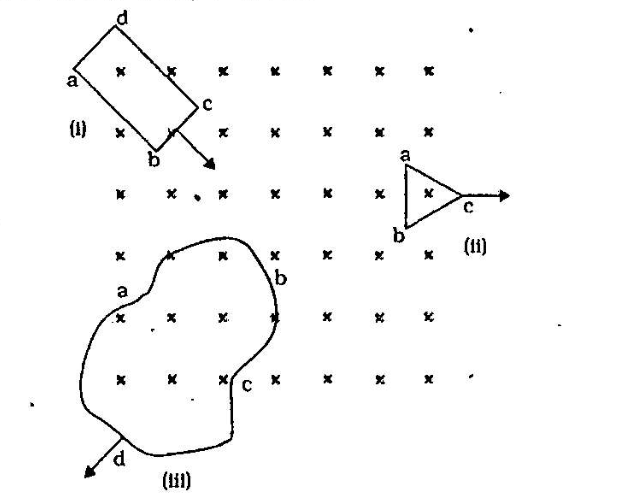Home/Class 12/Physics/

The figure below shows planar loops of different shapes moving out of or into a region of a magnetic field which is directed normal to the plane of the loop away from the reader. Determine the direction of induced current in each loop using Lenz’s law.Speed
00:00
04:13## QuestionPhysicsClass 12

The figure below shows planar loops of different shapes moving out of or into a region of a magnetic field which is directed normal to the plane of the loop away from the reader. Determine the direction of induced current in each loop using Lenz’s law.See the analysis below.
4.64.6## Solution

In figure $$(i)$$, the rectangular loop is going into the magnetic field region, so that the flux through the loop increases. Hence, according to Lenz’s law, the direction of induced current is such that it will oppose the cause which has induced it. So, the induced current must move along the path $$\text{abcda}$$ to oppose the increased flux.
In figure$$(ii)$$, the triangular loop moves out the magnetic field, so that the flux linked with the loop decreases. According to Lenz’s law, the induced current moves along the path $$\text{bacb}$$ to increase the cause which has induced it.
In figure$$(iii)$$, the arbitrary shaped loop moves out of the region of magnetic field, so that the flux linked with the loop decreases. Hence, according to Lenz’s law, the induced current is along the path $$\text{dabcd}$$ so that it can increase the flux.